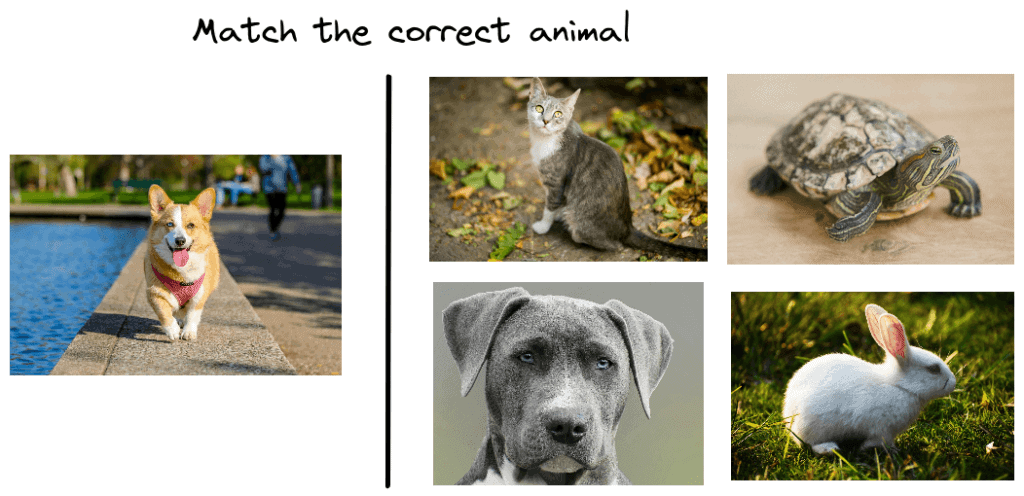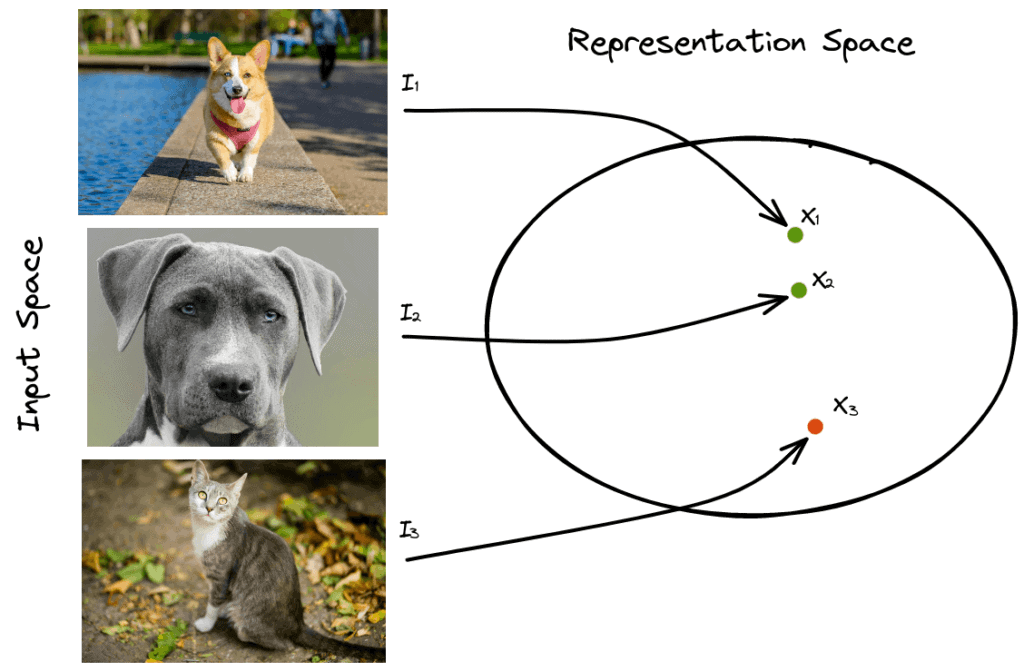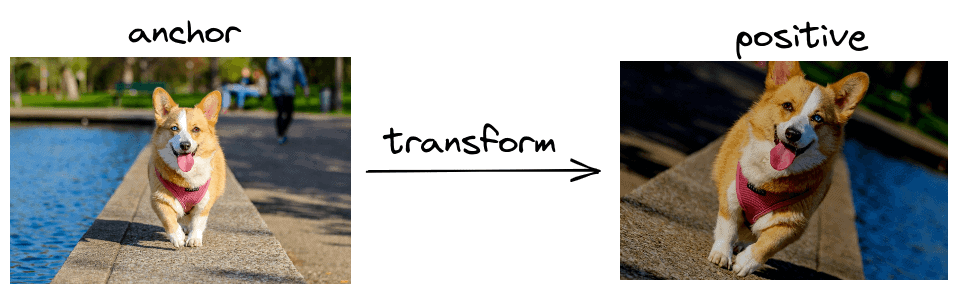## 1. Overview

In this tutorial, we’ll introduce the area of contrastive learning. First, we’ll discuss the intuition behind this technique and the basic terminology. Then, we’ll present the most common contrastive training objectives and the different types of contrastive learning.

## 2. Intuition

First, let’s talk about the intuition behind contrastive learning. Below, we can see a traditional game that many kids play:The goal of this game is to look at the pictures on the right side and search for an animal that looks like the one on the left side. In our case, the kid has to search for a picture of a dog among the four pictures on the right. First, the kid has to compare each one of the four animals with a dog and then conclude that the bottom left image depicts a dog.

According to many surveys, kids learn more easily new concepts in this way than reading a book about animals. But why does this method work better?

It turns out that it is easier for someone with no prior knowledge, like a kid, to learn new things by contrasting between similar and dissimilar things instead of learning to recognize them one by one. At first, the kid may not be able to identify the dog. But after some time, the kid learns to distinguish the common characteristics among dogs, like the shape of their nose and their body posture.

## 3. Terminology

Inspired by the previous observations, contrastive learning aims at learning low-dimensional representations of data by contrasting between similar and dissimilar samples. Specifically, it tries to bring similar samples close to each other in the representation space and push dissimilar ones to be far apart using the euclidean distance.

Let’s suppose that we have three images,and. The first two images depict a dog, and the third image depicts a cat, and we want to learn a low-dimensional representation for each image (,and):In contrastive learning, we want to minimize the distance between similar samples and maximize the distance between dissimilar samples. In our example, we want to minimize the distanceand maximize the distancesandwhereis a metric function like euclidean.

The sample that is similar to the anchor sample () is defined as a positive sample () and the one that is dissimilar as negative ().

## 4. Training Objectives

The most important part of contrastive learning is the training objective that guides the model into learning contrastive representations. Let’s describe the most common objectives.

### 4.1. Contrastive Loss

Contrastive loss is one of the first training objectives that was used for contrastive learning. It takes as input a pair of samples that are either similar or dissimilar, and it brings similar samples closer and dissimilar samples far apart.

More formally, we suppose that we have a pairand a labelthat is equal to 0 if the samples are similar and 1 otherwise. To extract a low-dimensional representation of each sample, we use a Convolutional Neural Networkthat encodes the input imagesandinto an embedding space whereand. The contrastive loss is defined as:whereis a hyperparameter, defining the lower bound distance between dissimilar samples.

If we analyze in more detail the above equation, there are two different cases:

• If the samples are similar (), then we minimize the termthat corresponds to their Euclidean distance.
• If the samples are dissimilar (), then we minimize the termthat is equivalent to maximizing their euclidean distance until some limit.

### 4.2. Triplet Loss

An improvement of contrastive loss is triplet loss that outperforms the former by using triplets of samples instead of pairs.

Specifically, it takes as input an anchor sample, a positive sampleand a negative sample. During training, the loss enforces the distance between the anchor sample and the positive sample to be less than the distance between the anchor sample and the negative sample:When we train a model with the triplet loss, we require fewer samples for convergence since we simultaneously update the network using both similar and dissimilar samples. That’s why triplet loss is more effective than contrastive loss.

## 5. Types of Learning

The idea of contrastive learning can be used in both supervised and unsupervised learning tasks.

### 5.1. Supervised

In this case, the label of each sample is available during training. So, we can easily generate positive and negative pairs or triplets by just looking into the labels.

However, generating all possible pairs or triplets requires a lot of time and computational resources. Also, in every dataset, there are many negative pairs or triplets that already satisfy the contrastive training objectives and give zero loss resulting in slow training convergence.

To deal with this problem, we have to generate hard pairs and hard triplets, meaning that their loss value is high, i.e., similar pairs that are far apart and dissimilar pairs that are very close. Many hard negative mining methods have been proposed that usually look into the representation space for hard pairs and triplets using fast search algorithms. In natural language processing, a simple way of generating a hard negative pair of sentences is to add in the anchor sentence a negation word.

### 5.2. Unsupervised

When we don’t have labeled samples, self-supervised learning is used where we exploit some property of our data to generate pseudo-labels.

A famous self-supervised framework for unsupervised contrastive learning is SimCLR. Its main idea is to generate positive image pairs by applying random transformations in the anchor image like crop, flip and color jitter since these changes keep the label of the image unchanged:## 6. Conclusion

In this tutorial, we talked about contrastive learning. First, we presented the intuition and the terminology of contrastive learning, and then we discussed the training objectives and the different types.